## A new ansatz for extremal Laurent polynomials

This post describes a new method for generating Laurent polynomials in 3 variables.  Many of these Laurent polynomials are extremal or of low ramification, and they include the extremal Laurent polynomials mirror to 15 of the 17 minimal Fano 3-folds. We call this method the Minkowksi ansatz.

Let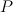$P$ be a 3-dimensional reflexive polytope.  We will construct a Laurent polynomial with Newton polytope equal to$P$, or in other words we will explain how to assign a coefficient to each integer point in$P$.  This goes as follows.

Lattice Minkowski sums

We say that a polygon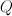$Q$ is the lattice Minkowski sum of polygons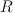$R$ and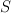$S$ if and only if both:

•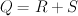$Q = R + S$, so that$Q$ is the Minkowski sum of$R$ and$S$ as usual
• the integer lattice in$Q$ is the sum of the integer lattices in$R$ and$S$.

Note that any of the the polygons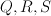$Q, R, S$ here are allowed to be degenerate.

Examples:  here are two lattice Minkowksi decompositions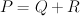$P = Q+R$ of a hexagon:

Note that the same lattice polygon can have more than one lattice Minkowski decomposition.  Note also that the first decomposition here is not a complete decomposition into lattice-Minkowksi-irreducible pieces, because the square$Q$ can be further decomposed as the sum of a vertical and a horizontal line.

The example above is not a lattice Minkowski decomposition, because the lattice in$P$ is not the sum of the lattices in$Q$ and$R$.  In fact$P$ is lattice Minkowski irreducible.

Decompose the facets into irreducible pieces

There are 4319 3-dimensional reflexive polytopes.  These polytopes contain a total of 344 distinct facets, where we regard two facets as the same if and only if they differ by a lattice-preserving automorphism.  Of these facets, 79 are lattice Minkowski irreducible.  These 79 facets are also the non-degenerate polygons which occur when the 344 total facets are decomposed into lattice Minkowksi irreducible pieces.  Of those 79 facets, exactly 8 contain no interior lattice points.  Those 8 triangles, which we call admissible triangles are all of type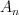$A_n$:

In other words, the cones over these triangles give affine toric varieties that are transverse$A_n$ singularities, for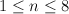$1 \leq n \leq 8$.

The ansatz

Given a 3-dimensional reflexive polytope$P$, we construct a possibly-empty list of Laurent polynomials as follows.  For each facet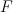$F$ of$P$, decompose$F$ into lattice-Minkowksi-irreducible pieces in all possible ways.  Discard any such decomposition of$F$ which contains a non-degenerate polygon that is not an admissible triangle.  Any remaining decomposition of$F$ will consist of line segments and admissible triangles.  To this decomposition we associate a Laurent polynomial which is the product of certain basic Laurent polynomials corresponding to line segments and  to admissible triangles.  The basic Laurent polynomials for admissible triangles are:

and so on for the remaining admissible triangles.  The basic Laurent polynomials for line segments are:

and so on for other line segments.

So now, for each facet$F$ of$P$, we have a list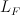$L_F$ of Laurent polynomials; this list will be empty if$F$ cannot be written as a lattice Minkowksi sum of line segments and admissible triangles. In other words for each facet$F$ we have list of ways of assigning coefficients to each integer point in$F$.  We seek a list of Laurent polynomials with Newton polytope equal to$P$, or in other words a list of ways of assigning coefficients to each integer point in$P$.  This is produced by assigning the coefficient zero to the origin (which is the only interior point of$P$) and then assigning coefficients to the integer points on facets of$P$ as specified in the facet lists (but amalgamated in all possible ways, so if there are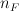$n_F$ elements in the list for facet$F$ then the number of elements in the list for$P$ is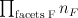$\prod_{\text{facets F}} n_F$).

Points to Note

• This ansatz almost generalizes the earlier recipes given by Pryjzalkowski and Galkin, but differs a little because of the difference between Minkowski decomposition and lattice Minkowksi decomposition.
• Altman has studied the deformation theory of affine toric varieties and discovered a close connection with Minkowski decompositions.  Since we expect to find the local system associated to an extremal Laurent polynomial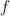$f$ as a piece of the quantum cohomology local system associated to a smoothing of the Newton polytope of$f$, this is encouraging.  But note that Minkowski decomposition and lattice Minkowksi decomposition are not the same.
• We suspect that if$P$ is a 3-dimensional reflexive polytope containing a facet with no admissible lattice Minkowski decompositions then the toric variety corresponding to$P$ does not smooth.  More on this later.
• This ansatz also fits well with  Kouchnirenko’s criterion for a Laurent polynomial to be degenerate.

(I learned this last point from Hiroshi.)

1.Sergei says:

We suspect that if is a 3-dimensional reflexive polytope containing a facet with no admissible lattice Minkowski decompositions then the toric variety corresponding to does not smooth.

You mean the singularity corresponding to the cone over this facet does not admit a smoothing?

2.Sergei says:

The ansatz looks reasonable, since it obviously respects the simplest type of the symplectomorphisms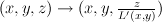$(x,y,z) \to (x,y,\frac{z}{L'(x,y)})$

where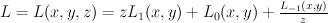$L = L(x,y,z) = z L_1(x,y) + L_0(x,y) + \frac{L_{-1} (x,y)}{z}$

and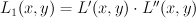$L_1(x,y) = L'(x,y) \cdot L''(x,y)$

the product corresponds to some Minkowski decomposition of the facet supporting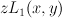$z L_1(x,y)$ i.e.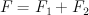$F = F_1 + F_2$ and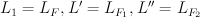$L_1=L_F, L'=L_{F_1}, L''=L_{F_2}$.

I expect there is an ansatz like that for all smoothable polytopes (maybe not Gorenstein).

3.Tom says:

Note that my original post contained a mistake which I have now corrected: the only change is the description of how to go from a collection of lists of Laurent polynomials, one for each facet, to a list of Laurent polynomials for the polytope$P$.

Sergei: does your comment 2 still apply to this corrected version?

4.Tom says:

@Sergei: with regard to comment 1, what Alessio expects is that if a 3d reflexive polytope$P$ contains a face$F$ without admissible decompositions then the toric variety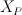$X_P$ corresponding to$P$ is not smoothable. This is precisely because, as you suggest, he expects that the toric co-ordinate patch on$X_P$ corresponding to the cone over$F$ will not smooth. But even if each face of$P$ is such that the cone over it gives a smoothable affine toric variety, I expect that there may still be some global obstruction to smoothability because the local smoothings (i.e. the smoothings of the toric co-ordinate patches) may not glue together to give a smoothing of the whole toric variety.

5.Sergei says:

I cannot see what was the typo, but my comment2 is a positive feedback to the ansatz you wrote now — if there are two parallel facets, you can transmit some of their divisors between them.

6.Sergei says:

On local-to-global obstructions to smoothability: in dimension 2 they vanish, for ODP’s in dimension 3 they also vanish.

There are some questions:

Q1. a) What are the smoothable normal 3-dimensional toric singularities?
There are some isolatedand some germs of non-isolated
b) What are the possible incidence relations between them?

Q2. Are local-to-global obstructions to smoothability vanish for smoothable toric singularities in dimension 3?

Q3. Show (or disprove) that
if
the affine singularities corresponding to the cones over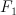$F_1$ and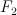$F_2$ are smoothable
then
the affine singularity corresponding to the cone over the Minkowski sum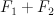$F_1 + F_2$ is smoothable?

According to Prokhorov Q1 is open.
Answer for Q3 could be in Altmann-Straten.

7.Tom says:

I corrected a typo (343 distinct facets->344 distinct facets, twice) pointed out by Al. Sorry!